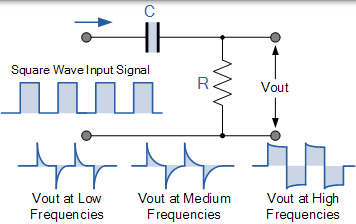# 无源高通滤波器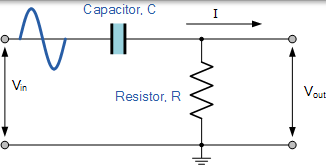## 高通滤波器电路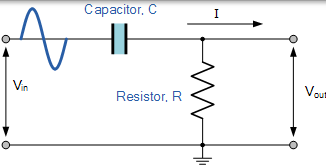### 一阶高通滤波器的频率响应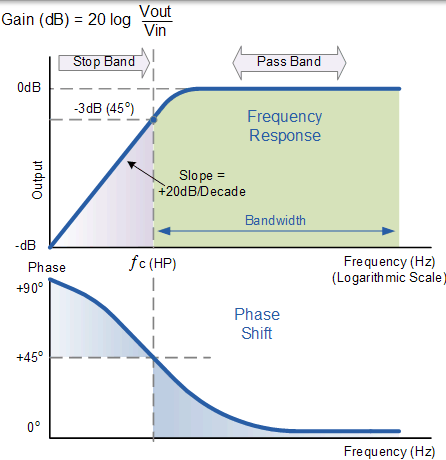### 截止频率和相移

$$\begin{array} { l } { f c = \frac { 1 } { 2 \pi R C } } \\ { \text { Phase Shift } \phi = \arctan \frac { 1 } { 2 \pi f R C } } \end{array}$$

$$A _ { v } = \frac { V _ { O U T } } { V _ { I N } } = \frac { R } { \sqrt { R ^ { 2 } + X c ^ { 2 } } } = \frac { R } { Z }$$

## 高通滤波器示例 No1

$$f c = \frac { 1 } { 2 \pi R C } = \frac { 1 } { 2 \pi \times 240,000 \times 82 \times 10 ^ { - 12 } } = 8,087 H z \text { or } 8 k H z$$

## 二阶高通滤波器

### 二阶高通滤波器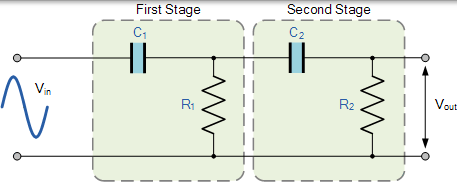$$f _ { \mathrm { C } } = \frac { 1 } { 2 \pi \sqrt { \mathrm { R } _ { 1 } \mathrm { C } _ { 1 } \mathrm { R } _ { 2 } \mathrm { C } _ { 2 } } } \mathrm { Hz }$$

## RC 微分器

### RC 微分电路